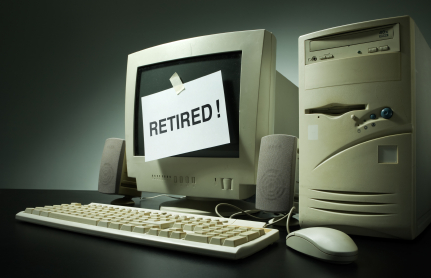# Accounting – Straight-line Method of DepreciationDepreciation is a systematic reduction in the cost of a fixed asset in your business. As fixed assets such as machines wear out, they tend to lose value. Think about the computer you have been using. Perhaps you expect it to serve you for about six years. Do you expect it to have the same value as you bought it at the end of six years? Note that every time you use it, its components get used up a little bit and the ever changing technology will one day make it obsolete. This ‘using up’ is known as depreciation.

Straight line depreciation is a common approach to matching your business’s assets to the accounting periods in which the assets were in use. Under this method of depreciation, you should reduce the cost of a fixed asset uniformly over the asset’s useful life. Since you will charge the same amount of depreciation expense to income statement in each accounting period, you will notice that the carrying amount of the asset being depreciated will decline on a straight line.

This method of depreciation is simple and is used by many businesses. Note that the accounting principles require businesses to depreciate their assets using a method that clearly reveals the pattern in which the asset is getting used up. Though straight line method of depreciation is effective, some assets tend to lose more value in their initial years of use. In such cases, you may need to use other methods of depreciation.

How to calculate depreciation expense

According to straight line depreciation method, you can determine the amount of depreciation expense in each accounting period by dividing the depreciable value of the asset by the asset’s useful life.

So, depreciation = Depreciable amount ÷ the asset’s useful life

while depreciable amount = cost of the asset – salvage value

Note that salvage value is also known as scrape value or residual value. It is the estimated value of the fixed asset at the end of its useful period. This useful period represents the number of accounting periods within which you expect the asset to generate economic benefits.

In some cases, the acquisition of an asset doesn’t coincide with the beginning of a financial year. In such a case, you may need to charge proportionate depreciation for the partial year.

When recording depreciation expense in your accounting books, debit the depreciation account and credit the same amount to the accumulated depreciation account. In any case, if you are not confident enough to record such entries, you can consider leveraging on accounting services in Singapore so to ensure things are on track.